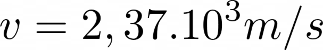Mechanics

# Escape velocity

The escape velocity is the minimum necessary velocity that an object needs to have in order to escape the gravitational attraction of a celestial body.

The escape velocity (V e ) is the minimum velocity that a body needs to obtain in order to escape the gravitational field exerted by celestial bodies. When launching objects from the surface, they are drawn back to the ground due to the gravitational pull. However, it is possible to reach a minimum velocity that can overcome this gravitational pull, which we call escape velocity.

This speed is demonstrated when the total mechanical energy (E m ) of the body when launched into infinity is zero. The escape velocity does not depend on the mass of the object trying to escape , but is related to the mass of the planet or star (M) from which one wants to escape, with the radius of that celestial body (R) and with the constant of universal gravitation ( G), which has a value of 6.67.10 -11 Nm 2 /kg 2 .

## Escape Velocity Equation

When an object manages to escape the gravitational attraction, we consider that it reaches infinity, so its kinetic energy (K), associated with the agitation of the particles of the body, and its potential energy (U), associated with the position that the bodies occupy, are final. null.

According to the law of conservation of energy , an isolated system interacts only with conservative forces and thus the total energy of the system is constant and not lost, so the total energy of the projectile is given by the following equation:On what:

• m: mass of the object;
• M: mass of the celestial body;
• v: object speed;
• R: radius of the planet;
• G: gravitational constant.

By isolating the velocity, we were able to exclude the object’s mass: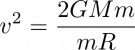So, to find the escape velocity, we arrive at the following formula: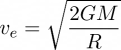With this equation, you can find the minimum speed a body needs to have to escape a planet’s gravitational field.

Let’s exemplify the use of the formula considering the case of Earth :

• G = 6.67.10 -11 Nm 2 /kg 2
• M = 5.98.10 24 kg
• R = 6.38.10 6 m## Escape velocity from other planets

 stars Mass (kg) radius (m) Escape velocity (km/s) Sun 1.99.10 30 6.96.10 8 617.54 Mercury 3.30.10 23 2.43.10 6 4.3 Venus 4.86.10 24 6.05.10 6 10.4 Moon 7.36.10 22 1.74.10 6 2.4 Mars 6.41.10 23 3.38.10 6 5.1 Jupiter 1.90.10 27 7,15,10 7 61 Saturn 5.68.10 26 5.82.10 7 36.7 Uranus 8.68.10 25 2.53.10 7 22.4 Neptune 1.02.10 26 2.46.10 7 25.5

## Orbital Velocity Escape Velocity

Be careful not to confuse these two speeds!

• Escape Velocity: Minimum velocity to escape a planet’s gravitational pull.
• Orbital speed: speed at which a celestial body describes its orbit.

Johannes Kepler defined three laws to describe the motion of planets in the Solar System. Its 3rd law describes that the farther this celestial body is in relation to the Sun (size of its orbit), the lower its speed of translation (time to go around the sun).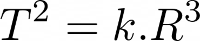• T: period of revolution of the celestial bodies.
• K: proportional constant of the star that the celestial bodies orbit.

To find the orbital velocity, it is necessary to compare the law of universal gravitation with the equation of centripetal force , the resultant force that points towards the center of motion and is exerted on the celestial body. So, we will have:By cutting the mass of the celestial body (m) and the radius (R), which appear on both sides of the equation, we are able to isolate the orbital velocity:## Exercises solved

Question 1 – (Fuvest 2020) The escape velocity of a celestial body is the minimum velocity that an object must have near the surface of that body to escape its gravitational attraction. Based on this information and your knowledge of the kinetic interpretation of temperature, consider the following statements regarding the relationship between the escape velocity and the atmosphere of a celestial body.

I. Celestial bodies with the same escape velocity retain equally dense atmospheres, regardless of the temperature of each body.

II. Nitrogen gas molecules escape from the atmosphere of a celestial body more easily than hydrogen gas molecules.

III. Comparing celestial bodies with equal average temperatures, the one with the highest escape velocity tends to retain a denser atmosphere.

Only what is said in:

THERE.

B) II.

C) III.

D) I and II.

E) I and III

Resolution

Alternative C.

The planet’s temperature affects the density of its atmosphere. So the higher the temperature, the lower the density of the atmosphere and vice versa.

Lighter (less dense) bodies have a greater facility to escape from celestial bodies, as their kinetic energy is lower. Thus, only alternative III is correct, since nitrogen is heavier than hydrogen and temperature changes the density.

Question 2 – The minimum speed for a body to leave the surface of a celestial body is called escape velocity. Determine the escape velocity for a probe to escape the surface of the Moon, in m/s.

Data:

• Moon Mass: 7.36.10 22
• Universal gravitation constant: 6.67.10 -11 Nm 2 /kg 2

A) 5.64.10 6

B) 2.37.10 3

C) 2.82.10 6

D) 3,13,10 6

Resolution

Alternative B.

To find the escape velocity, we will use the following equation :Substituting with the information presented in the statement:Solving the multiplication :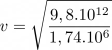Solving the division :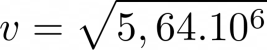Taking the value from the root: